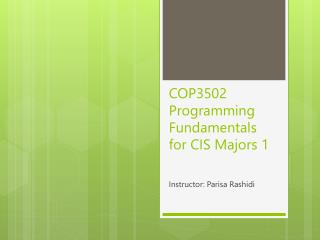DownloadDownload PresentationCOP3502 Programming Fundamentals for CIS Majors 1

# COP3502 Programming Fundamentals for CIS Majors 1

Download Presentation## COP3502 Programming Fundamentals for CIS Majors 1

- - - - - - - - - - - - - - - - - - - - - - - - - - - E N D - - - - - - - - - - - - - - - - - - - - - - - - - - -
##### Presentation Transcript

1. COP3502 Programming Fundamentals for CIS Majors 1 Instructor: Parisa Rashidi

2. Chapter 6 • Array • Single dimensional array • Search & sort

3. Chapter 7 • Multi-dimensional arrays • Declare • Create • Access • Problem solving using two dimensional array • Sudoku • …

4. Multi-Dimensional Arrays

5. // Declare array refVar of type dataType dataType[][] refVar; // Create array refVar = newdataType[size1][size2]; • Declaring and creating 2-dimensional array • Example // Declare array double[][] myTable; // Create array myTable= newdouble;

6. // Combine declaration and creation in one // single statement double[][] myTable= newdouble; // Alternative syntax doublemyTable[][] = newdouble; • Declaring and creating 2-dimensional array

7. int[][] matrix = new int • 2-dimensional array illustration • Declaring and creating an array 0 1 2 3 4 0 1 2 3 4

8. final intBLANK = 0; // location empty final intWHITE = 1;// white piece final intRED = 2; // blue piece int[][] checkerBoard= new int • We want to store the position on a checkers’ board.

9. String[] pieces = {“king”, “queen”, “rook”, “knight”, “bishop”, “pawn”}; int[][] chessBoard= new int • We want to store the position on a chess board.

10. matrix =7; • 2-dimensional array illustration • Accessing elements 0 1 2 3 4 0 1 2 3 4

11. int [][] nums = new int; • Internally, java stores a 2D array as “an array of arrays” nums

12. What is nums.length? • 5 • What is nums.length? • 4 int [][] nums = new int; nums

13. int[][] matrix2 = { {1, 2, 3}, {10, 3, 0}, {10, 7, 80} }; 0 1 2 • 2-dimensional array illustration • Initialization 0 1 2 matrix2

14. int[][] matrix = { {1, 2, 3, 4, 5}, {2, 3, 4, 5}, {3, 4, 5}, {4, 5}, {5} }; • Rows might have different lengths matrix

15. for (int row = 0; row < matrix.length; row++) { for (int column = 0; column < matrix[row].length; column++) { // use matrix[row][column] } } • Loops and 2D arrays

16. java.util.Scanner input = new Scanner(System.in); System.out.println("Enter " + matrix.length + " rows and " + matrix.length + " columns: "); for (int row = 0; row < matrix.length; row++) { for (int column = 0; column < matrix[row].length; column++) { matrix[row][column] = input.nextInt(); } } • Initializing 2D array with input values

17. for (int row = 0; row < matrix.length; row++) { for (int column = 0; column < matrix[row].length; column++) { matrix[row][column] = (int)(Math.random() * 100); } } • Initializing 2D arrays with random values

18. for (int row = 0; row < matrix.length; row++) { for (int column = 0; column < matrix[row].length; column++) { System.out.print(matrix[row][column] + " "); } System.out.println(); } • Printing 2D arrays

19. int total = 0; for (int row = 0; row < matrix.length; row++) { for (int column = 0; column < matrix[row].length; column++) { total += matrix[row][column]; } } • Summing all elements of a 2D array

20. for (int column = 0; column < matrix.length; column++) { int total = 0; for (int row = 0; row < matrix.length; row++) total += matrix[row][column]; System.out.println("Sum for column " + column + " is " + total); } • Summing elements of a 2D array by each column

21. for (inti = 0; i < matrix.length; i++) { for (int j = 0; j < matrix[i].length; j++) { int i1 = (int)(Math.random() * matrix.length); int j1 = (int)(Math.random() * matrix[i].length); // Swap matrix[i][j] with matrix[i1][j1] int temp = matrix[i][j]; matrix[i][j] = matrix[i1][j1]; matrix[i1][j1] = temp; } } • Random shuffling of a 2D array

22. Passing 2D array to a method PassTwoDimensionalArray Run